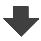Search:

# Displacement of the chemical equilibrium

## Iron(III) and thiocyanate

Recall the equation of this equilibrium!

$Fe^{3+}$ $+$ $SCN^-$ $\rightleftarrows$ $Fe(SCN)^{2+}$ (disregarding the hydration of ions)

Why does the addition of $KCl$ shift the equilibrium to the left (merely visible)?

According to the principle of le Châtelier, the addition of a product tends to remove a portion of the products to reform the reactants.

Why does the addition of $Fe^{3+}$ or $SCN^-$ shift the equilibrium to the right?

According to the principle of le Châtelier, the addition of a reagent tends to remove some of this reagent to form products.